Get inspired by the success stories of our students in IIT JAM MS, ISI  MStat, CMI MSc DS.  Learn More

# ISI MStat 2016 (Sample) Problem 2 | Continuous function | PSBThis is a beautiful sample problem from ISI MStat 2016 PSB Problem 2. This is based on application of continuity and integration.

## Problem- ISI MStat 2016 Problem 2

Let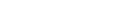be a continuous function. Suppose that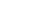exists and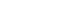for all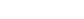. If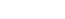and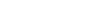prove that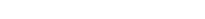.

## Solution

Given function is continuous andexists .

Hence we can integrate.

Another thing is given thatfor all----(1) (say)

Now if we integrate both side of (1) from -1 to y , where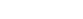then we get ,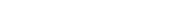, for all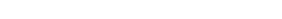,for all[ since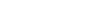given ]---(2)

Again if we integrate both side of (1) from y to 1 ,wherethen we get,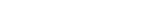, for all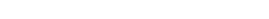,for all[ sincegiven ] ---(3)

Hence from (2) & (3) we get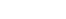for alland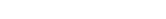.

Therefore ,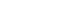for all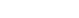( proved )

## Challenge Problem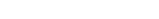be continuous function such that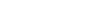for every x and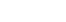Then find f(x) .

This is a beautiful sample problem from ISI MStat 2016 PSB Problem 2. This is based on application of continuity and integration.

## Problem- ISI MStat 2016 Problem 2

Letbe a continuous function. Suppose thatexists andfor all. Ifandprove that.

## Solution

Given function is continuous andexists .

Hence we can integrate.

Another thing is given thatfor all----(1) (say)

Now if we integrate both side of (1) from -1 to y , wherethen we get ,, for all,for all[ sincegiven ]---(2)

Again if we integrate both side of (1) from y to 1 ,wherethen we get,, for all,for all[ sincegiven ] ---(3)

Hence from (2) & (3) we getfor alland.

Therefore ,for all( proved )

## Challenge Problembe continuous function such thatfor every x andThen find f(x) .

This site uses Akismet to reduce spam. Learn how your comment data is processed.

### Knowledge Partner